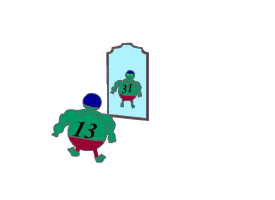Problems

# "Mirror prime" numbers

We will call a number "mirror prime", if it is prime, and the number written in a reverse order is also prime.

Find the number of "mirror primes" from a to b.#### Input

Two integers a and b (1ab10000).

#### Output

Print the number of "mirror primes" from a to b inclusive.

Time limit 1 second
Memory limit 128 MiB
Input example #1
10 25

Output example #1
3